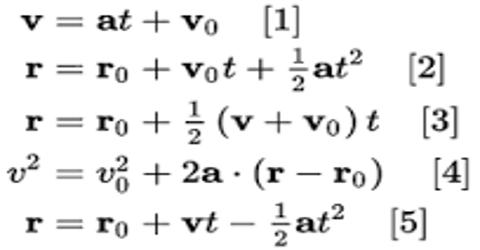# Fourth Equation of Dimensional Motion

Fourth Equation

Relation between initial velocity, final velocity and displacement or position

v2 = v02 + 2as or, vx2 = vx02 + 2a (x – x0)

Let an object move at a particular direction with uniform acceleration ‘a’. Let at t = 0 its initial velocity be v0 and at t = t, the final velocity ‘v’ and during this time interval the displacement of the object is ‘s’. Now, we know,

v = v0 + at … … … (1)

and s = v0t + ½ at2 … … … (2)

Now squaring both sides of equation (1), we get;

v2 = (v0 + at)2 = v02 + 2 v0at + a2t2

= v02 + 2a (v0t + ½ at2)

= v02 + 2as [using equation (2)] … … … (3)

For one dimensional notion, say along X-axis let v0 = vx0, v = vx, a = ax and s = x – x0, where x0 is the initial position at t = 0 and x the final position at t = t. Then from equation (3) we get;

vx2 = vx02 + 2ax + 2ax (x – x0)